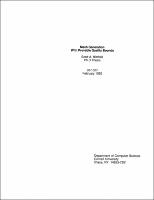## Mesh Generation With Provable Quality Bounds##### Abstract
We consider the problem of generating a triangulation of provable quality for two and three dimensional polyhedral regions. That is, we seek a triangulation, allowing additional vertices called Steiner points, such that the triangular or tetrahedral elements have a bound on their shape. In three dimensions we also seek an upper bound on the number of tetrahedra in the triangulation. These triangulation algorithms find application in mesh generation for finite element methods. The polyhedral region must be bounded and well defined, but may have holes of arbitrary complexity. In three dimensions, we assume there are no restrictions on where we may place Steiner points. Our triangulation is optimal in the following two senses. First, our triangulation achieves the best possible aspect ratio up to a constant factor, which is our bound on element shape. Second, for any other triangulation of the same region into $m$ triangles with bounded aspect ratio, our triangulation has size $n = O(m)$. Such a triangulation is desired as an initial mesh for a finite element mesh refinement algorithm. Previous three dimensional triangulation schemes either worked only on a restricted class of input, or did not guarantee well-shaped tetrahedra, or were not able to bound the output size. We build on some of the ideas presented in previous work by Bern, Eppstein and Gilbert, who have shown how to triangulate a two dimensional polyhedral region with holes, with similar quality and optimality bounds. In two dimensions, we assume the restriction that we may introduce Steiner points on the polygon's interior, but not on its boundary. Of all triangulations satisfying this restriction, our triangulation has the maximum minimum angle, up to a constant factor. This is the first known algorithm for this problem with provably optimal element shape. The algorithm solves several subproblems of mesh generation.
1993-02
##### Publisher
Cornell University
##### Keywords
computer science; technical report
##### Previously Published As
http://techreports.library.cornell.edu:8081/Dienst/UI/1.0/Display/cul.cs/TR93-1327
technical report